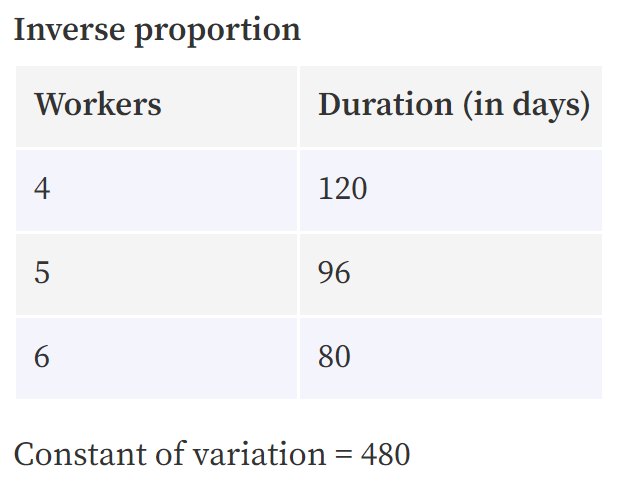# Result of the Day 1 [Problem A] Challenge of my July 2021 Math mini-contest for D.Buzz

in STEMGeeks4 months ago

## Day 1 Problem A

In a construction project, adding 1 worker will decrease the project duration by 24 days, while adding 2 workers will decrease duration by 40 days. What is the original number of workers and days?

The problem has originally been posted at https://stemgeeks.net/@savvyplayer/julymathday1a.

## days = 120

Both values must be correctly supplied on an answer for it to be considered correct.

## Solution

The problem is under elementary algebra.

When the number of workers for a project increases, the shorter the duration of the project will be. The solution will make use of the inverse proportion formula.

## y = k / x

where

x = number of workers
y = time needed
k = constant of variation

Equation 1: y = k / x, where k = y * x
Equation 2: (y - 24) = k / (x + 1), where k = (y - 24) * (x + 1)
Equation 3: (y - 40) = k / (x + 2), where k = (y - 40) * (x + 2)

We can now combine Equation 2 with 1 and Equation 3 with 1 by replacing the k variables with their equivalents in terms of x and y.

Equation 4: yx = (y - 24) * (x + 1) = yx + y - 24x - 24
Equation 5: yx = (y - 40) * (x + 2) = yx + 2y - 40x - 80

Combining the similar terms and arranging the remaining terms to be in slope-intercept form, we get:

Equation 4: y = 24x + 24
Equation 5: 2y - 40x = 80 which can be simplified to y = 20x + 40

It is clear that we have a system of linear equations in two variables. We can use the substitution method to get the value of x first.

Equation 6: 24x + 24 = 20x + 40, where we can obtain the value of x which is 4.
Equation 7: y = 24 * (4) + 24, where we can obtain the value of y which is 120.

Therefore, the original number of workers is 4, and the original duration of the project is 120 days.

WorkersDuration (in days)
4120
596
680

To make sure that our answer is correct, we should multiply the workers by the duration for each equation, where we would get 480 as the constant of variation for all of them.## Winner: none

1 HIVE has been transferred to the prize pool, which will be awarded to the participant with the highest number of correctly-answered problems after all the challenge problems in this Math mini-contest have been concluded.

Mentions: @jfang003, @holovision, @eturnerx (@eturnerx-dbuzz), @ahmadmanga (@ahmadmangazap), @appukuttan66, @paultactico2, @dkmathstats, and @minus-pi
Special mentions: @dbuzz, @chrisrice, @jancharlest, @lolxsbudoy, and @mehmetfix

Posted with STEMGeeks

Sort:

Wow